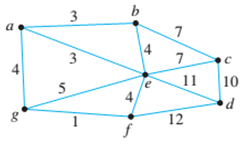Chapter 10.6, Problem 9ES### Discrete Mathematics With Applicat...

5th Edition
EPP + 1 other
ISBN: 9781337694193

#### Solutions

Chapter
Section### Discrete Mathematics With Applicat...

5th Edition
EPP + 1 other
ISBN: 9781337694193
Textbook Problem
3 views

# For each of the graphs in 9 and 10, find all minimum spanning trees that can be obtained using (a) Kruskal’s algorithm and (b) Prim’s algorithm starting with vertex a or t. Indicate the order in which edges are added to form each tree. 9.To determine

(a)

To find out all the minimum spanning trees that can be obtained using Kruskal’s algorithm.

Explanation

Given information:

The following is the given graph −

Calculation:

The Kruskal’s algorithm follows the following steps to form the minimum spanning tree from the given graph-

First Form-

1. Add the edge with least weight. In the given graph {g,f} is the edge with least weight so add it into the tree.
2. Now observe all the remaining edges and pick the edge with minimum weight and add it. Now there are two edges with minimum weights {a,b} and {a,e}, so add them also.
3. Now, observe the remaining edges. The edges {a,g} {b,e} {e,f} are the small edges with weight 4. Since the edge {b,e} forms the loop, it will never be added. So add the edge {a,g} to the tree.
4. Next add {e,c} and {c,d} to the graph.

Finally the following will be the edges in the minimum spanning tree obtained using Kruskal’s algorithm.

{g,f},{a,b},{a,e},{a,g},{e,c},{c,d}

Another minimum spanning tree can be formed.

Second Form-

Instead of adding the edge {c,e} to the tree, the edge {b,c} can be added with same weight to form the spanning tree in step 4

To determine

(b)

To find out all the minimum spanning trees that can be obtained using Prim’s algorithm.

### Still sussing out bartleby?

Check out a sample textbook solution.

See a sample solution

#### The Solution to Your Study Problems

Bartleby provides explanations to thousands of textbook problems written by our experts, many with advanced degrees!

Get Started